Multiple Choice questions on Magnetic field and magnetic effects of current for JEE Main and Advanced

Question 1
Find the force on the straight conductor of length .30 m carrying a current of 5 .0 A in the – k direction where the magnetic Field is given by  B=3.5 ×10-3 (i - j) T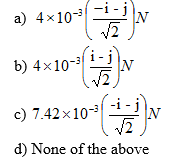Question 2
A current sheet having current K A/m is placed at in the x-y plane at z=0.The direction of current is -j
Find the Magnetic Field at on the z axis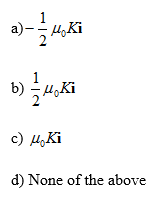Question 3
A current sheet where current per unit length is 6.0 A/m and direction is towards +i is placed at z=0 in the x-y plane. A current wire is located at y=0 and z=5 m. It is placed along the x axis. Find the current and its direction if Magnetic field is zero at the point P whose location is (0, 0, 2.5) m
a) 47 A, i
b) 47.1 A, -i
c) 50 A, i
d) 50 A, -i

Questions 4
A charged particle whose mass is 19.9 ×10-27 kg   and charge is 1.6×10-19 C moves with a speed of 3×105 m/s at right angle to a magnetic field of .75 T.
What is the force acting on the charge, centripetal acceleration and radius of the circle in which charged particle moves
a) 3.6×10-14   N,1.81×1012 m/s2, 49.7×10-3 m
b) 3.0×10-14   N,1.81×1012 m/s2, 49.7×10-3 m
c) 3.0×10-14   N,1.8×1012 m/s2, 49.7×10-3 mm
d) None of the above

Question 5
Which of the following is true?
A) Magnetic field exerts force only a moving charge.
B) Electric field exerts force on both stationary and moving charge
C) Magnetic field exerts force on charge moving parallel to the direction of the field
D) All the above

Question 6
Four Particles P1(proton), P2(electron), P3(alpha), P4(neutron) enters a region of constant magnetic field with same velocities. The magnetic field is perpendicular to the velocity
Match the following
Column A
A) what all particle will not be deflected by the Magnetic field
B) What all particles will be deflected by the Magnetic Field?
C) Which particle move in a circular path of maximum radius
D) Which particle will experience maximum Force?
Column B
P) P1
Q) P2
R) P3
S) P4

Question 7
The force experienced by a particle of charge q moving with a velocity v in a uniform magnetic field B is given by
F=q(vXB)
Statement I: The vectors F and v are perpendicular to each other
Statement II: The vectors v and B can have any angle between them
Statement III: The vectors F and B are perpendicular to each other
a) All the statements are true
b) Statement I and II are true only
c) Statement I and III are true only
d) Statement II and III are true only

Question 8
A long wire is bent as shown in figure. Find the magnitude and direction of the magnetic field at the center of the circular part if a current of I amp is passed through the wire
The different parts of the wire do not touch each other at Q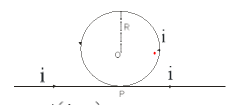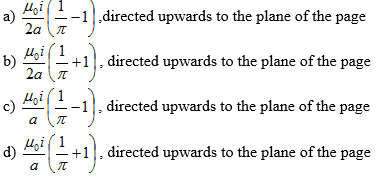Question 9
A non-conducting thin disc of Diameter D charged uniformly over one side with surface density  $\sigma$ rotates about its axis with an angular velocity $\omega$
What is the magnetic field at the center of the disc?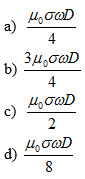Question 10
A charged particle moving with constant velocity passes through a space without any change in velocity
Electric Field in the region is given by the
E=a1i + a2 j + a3 k
Magnetic Field in the region is given by the
B=b1i + b2 j + b3 k
Where a1, a2, a3, b1, b2, b3 are constant
Which of the following can be true about the region?
a)   a1=a2=a3=b1=b2=b3 =0
b)  a1=a2=a3 =0, b1≠0 b2≠0 b3≠0
c) a1≠0 a2≠0 a3≠0, b1=b2=b3 =0
d) a1≠0 a2≠0 a3≠0 b1≠0 b2≠0 b3≠0

Question 11
A charged particle of mass m and charge q moves with a constant velocity along the positive x direction
v=a i.
it enters a region of Magnetic field which is directed towards positive z direction from x=a which is given by
B = b k
Find the initial acceleration of the particle
a) a=(-qab/m) j
b) a=(-qab/m) j
c) a=(-qa/mb) j
d) none of these

Question 12
Find the radius of the circular path which the particle moves
a) mb/qa
b) ma/q
c) mab/q
d) None of these

Question 13
Which of the following is true about the motion of the particle?
a) Force will always be perpendicular to the velocity
b) The magnitude of the velocity remains constant
c)  velocity vector and magnetic field vector remains perpendicular to each other during the motion
d) KE of the particle remains constants

Question 14
The frequency of the rotation
a) depends on the value a
b) depends on the value b
c) depends on the value a and b both
d) Does not depend on a and b both

Question 15
Four circular coil( A,B,C,D)  having  radius r,2r,3r,4r having numbers of turns  4n,3n,2n,n and carrying the same current   are there.
Match the Column
Column X
P) which coil will produce maximum Magnetic induction at the center
Q) which coil will produce minimum Magnetic induction at the center
R) Which coil has maximum magnetic moment per turn
S) Which coil has minimum magnetic moment per turn
Column Y
E) A
F) B
G) C
H) D

Question 16
An infinite long thin cylinder of radius R is carrying current I along the axial direction.
Which of the following is true?
a) B =0 for r< R
b)  B =k/r for r > R
c)  B=kr for r> R
d) none of the above

Note to our visitors :-

Thanks for visiting our website.
DISCLOSURE: THIS PAGE MAY CONTAIN AFFILIATE LINKS, MEANING I GET A COMMISSION IF YOU DECIDE TO MAKE A PURCHASE THROUGH MY LINKS, AT NO COST TO YOU. PLEASE READ MY DISCLOSURE FOR MORE INFO.